Want create site? Find Free WordPress Themes and plugins.

Topics In ECG: Step 1

Normal ECG
The electrocardiogram (ECG or EKG) is a diagnostic tool that is routinely used to assess the electrical and muscular functions of the heart. This article discusses the rate, rhythm, cardiac axis, and normal waves & intervals in an ECG.

Normal Sinus Rhythm

It refers to the rhythm that originates in the SA node.

ECG Findings:

• P wave precedes every QRS complex.
• Regular rhythm, but varies slightly during respiration
• Rate: 60–100 bpm
• The maximum amplitude of P waves is 2.5 mm in leads II and/or III.
• The P wave is positive in leads I and II and biphasic in lead V1.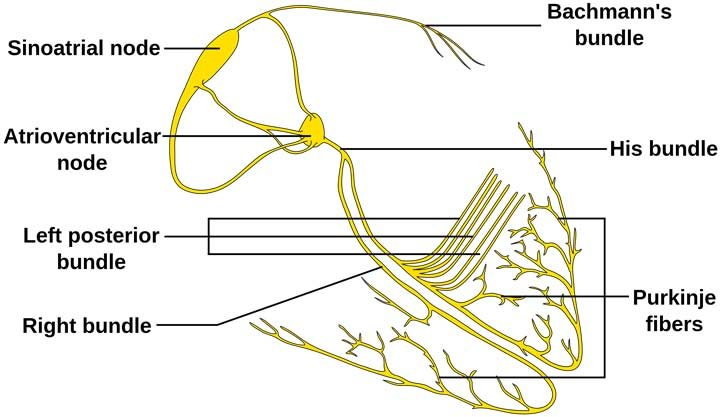Fig 2. Anatomy of the Conduction System.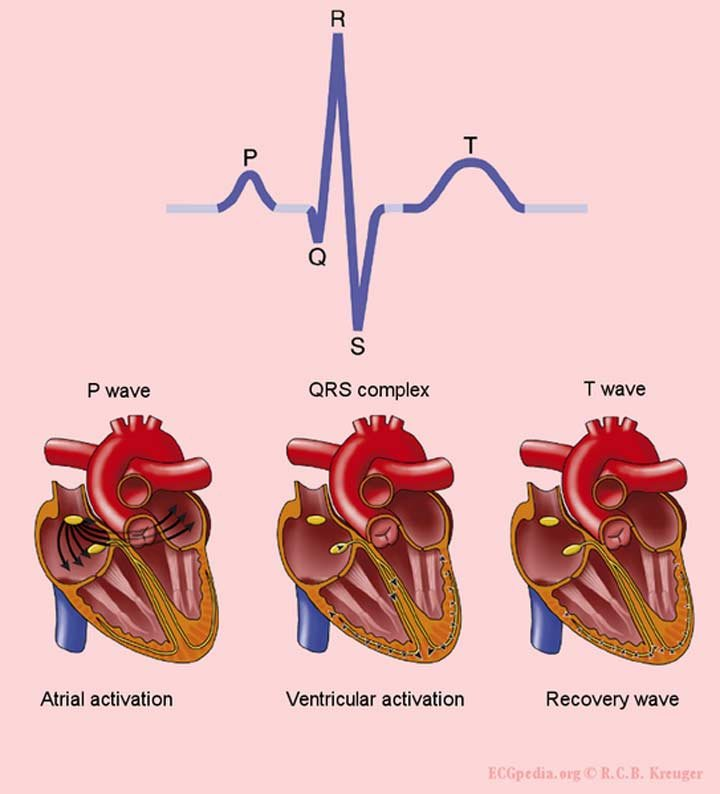Fig 3. Spread of depolarization through the conduction system.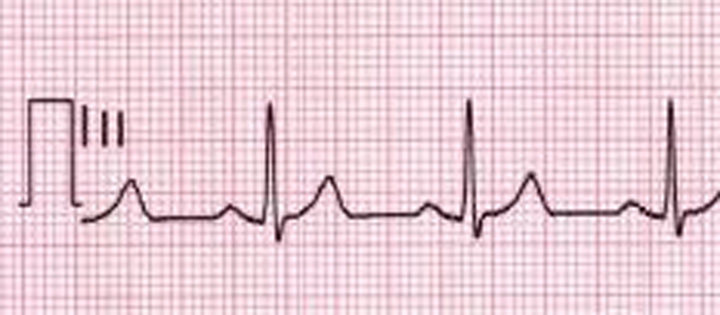Fig 4. ECG showing Sinus Rhythm.
Findings: Rate: 60–80 bpm, regular rhythm, an upright P wave preceding every QRS complex.

Normal Waves in ECG
P wave

• It is a small upward deflection representing atrial depolarization.
• It should be upright in leads I, II, aVF, and V2-V6 and inverted in aVR.
• P wave is normally upright, biphasic, flat, or inverted in V1, occassionally in lead V2.

QRS complex

• Q wave is a small downward deflection that follows P wave and represents depolarization of the interventricular septum.
• R wave is an upward deflection that usually follows Q wave.
• S wave is a small downward deflection that follows R wave and represents ventricular depolarization at the base of the heart.
• QRS is positive in all leads except aVR.

T wave

• It is an upward wave that follows the QRS complex.
• It represents ventricular repolarization.
• It is upright in all leads except aVR and V1.

U wave

• It is a small wave that follows T wave in the same direction.
• They are presumed to represent repolarization of the papillary muscles or Purkinje fibers.
• It is best seen in lead V2 and V3.
 Waves in ECG Normal Duration Normal Height P wave <120 ms <2.5 mm in limb leads, <1.5 mm in precordial leads QRS complex 40–100 ms <25 mm in any lead T wave 160 ms <5 mm in limb leads, <15 mm in precordial leads U wave 170–200 ms Maximum normal 1–2 mm

Normal Intervals and Segments in ECG

PR interval

• The time from the beginning of the P wave to the beginning of the QRS complex.
• Normal range ~ 120–200 ms.

PR segment

• From the end of the P wave to the beginning of the QRS complex.
• It is isoelectric and acts as a baseline to evaluate depression or elevation of the ST segment.
• Normal range ~ 50–120 ms.

QT interval

• Measured from the beginning of the QRS complex to the end of the T wave in leads II and V5-V6.
• It indicates the time taken for ventricular depolarization and repolarization.
• It is inversely proportional to the heart rate.

QTc interval/Corrected QT interval

• It estimates the QT interval at a heart rate of 60 bpm.
• This correction allows comparing QT intervals over time at different heart rates and also improves detection of patients at risk of ventricular arrhythmia.
• The most frequently used formula to estimate QTc interval is Bazett’s formula.
• Bazett’s formula states QTc = QT / √RR where QTc = Corrected QT interval, QT = Uncorrected QT interval, and RR = RR interval.
• The normal QTc interval is 390–450 ms in men and 390–460 ms in women.

ST segment

• It is the distance from the end of the S wave to the beginning of the T wave.
• It is usually isoelectric.
• Normal range: ~ 80–120 ms.

J point

• It is the point at which S wave joins the baseline.
• It is important in the evaluation of ST segment deviation like ST elevation or depression.

TP segment

• The portion between the end of T wave and the beginning of next P wave.
• At normal heart rate, it is usually isoelectric and used as a baseline for determination of deviation of the ST segment.

Ventricular activation time

• It is measured from the beginning of the Q wave to top of R wave.
• It should be <30 ms in leads V1-V2 and <50 ms in leads V5-V6.

Calculation of Heart Rates through ECG

The heart rate calculated through an ECG is typically the ventricular rate.

If atrial and ventricular rates differ, as in a third-degree block, we should measure both rates.

Regular rhythms:

• 1500 divided by the number of small squares between two R waves.
• 300 divided by the number of large squares between two R waves.

Irregular rhythms:

• Count the number of QRS complexes that fit into 3 s and multiply this number by 20 to determine the number of beats per minute (bpm).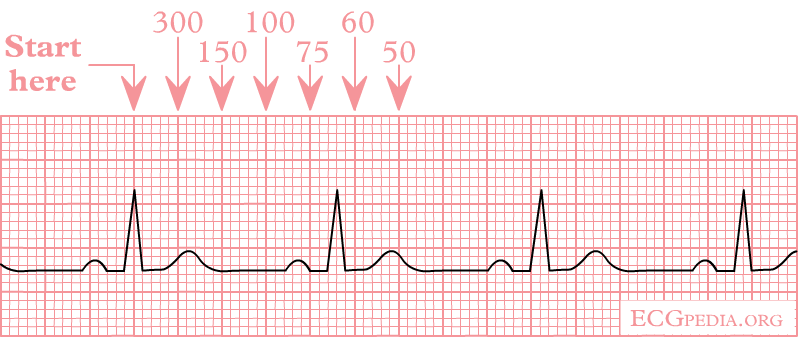Fig 1. ECG of an RR Interval for Calculation of the Heart Rate.

Cardiac Axis

• It is the average direction of the spread of depolarization wave through the ventricles as observed from front.
• The direction of axis can be derived easily from the QRS complexes in leads I, III, and aVF.
• In adults, the normal QRS axis is considered to be within -30° and +90°.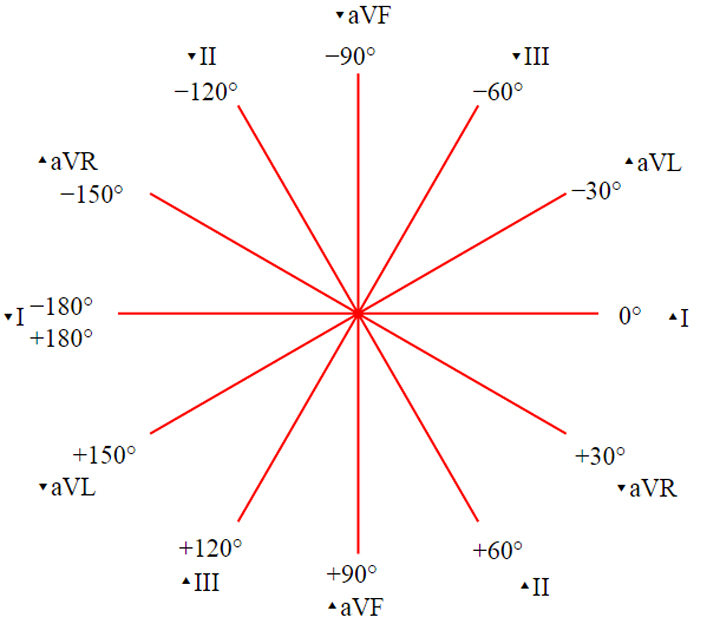Fig 5. The Hexaxial Reference System.

Interpretation of Cardiac Axis through ECG

 Lead I Lead aVF Axis Positive Positive Normal (00 to +900) Positive Negative Possible left axis deviation. Is lead II positive? Yes – Normal (00 to -300) No – Left axis deviation (-300 to -900) Negative Positive Right axis deviation (+900 to +1800) Negative Negative Extreme axis deviation (-900 to +1800)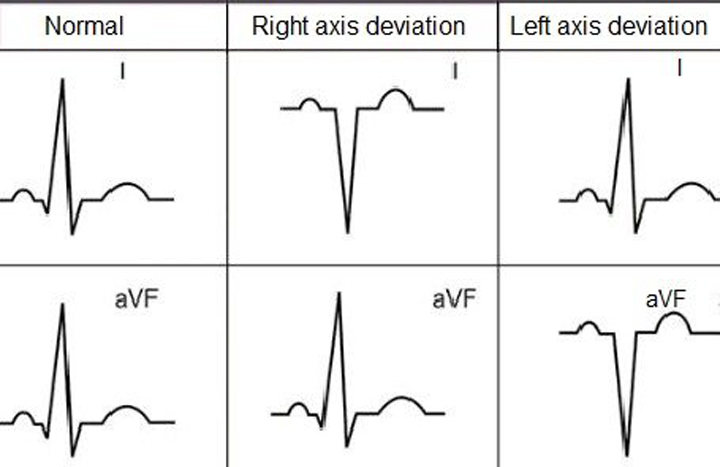Fig 6. Axis Deviation.

Rotation of Heart

• It is reflected in precordial leads.
• The QRS complex is generally isoelectric in leads V3 and V4.
• As a general rule, heart rotates towards hypertrophy and away from infarction.

Next Topic: Leads and Placement

Did you find apk for android? You can find new Free Android Games and apps.
error: Content is protected !!